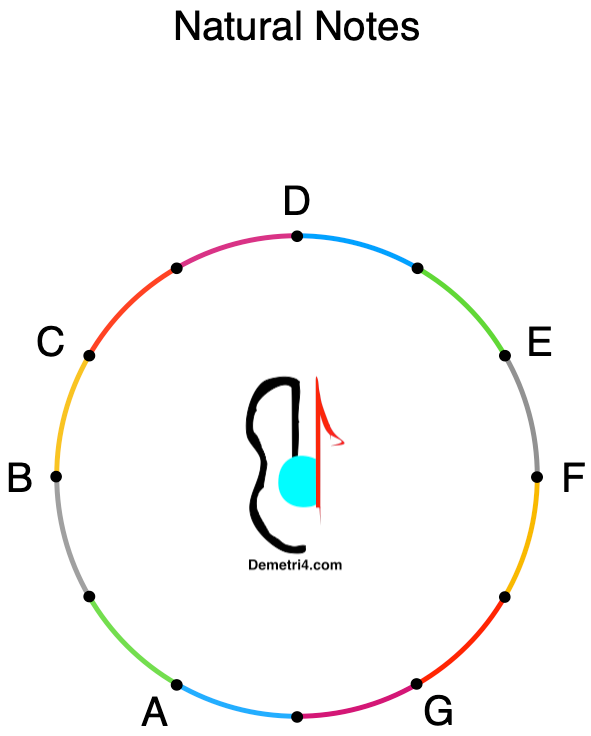top of page
Search
•Demetri Besougloff

# Blog #3: Why is the Tetra-chord so important?

Updated: Dec 20, 2022

For information about private lessons and questions, please visit me at Demetri4.com.

Here is the YouTube link to the video that goes with this blog: https://bit.ly/3VuAiEd

Blog #3

The Natural Notes

The Natural Notes are the notes in the C Major scale. All elements of music compare to the C Major scale. Therefore, we will refer to the C Major scale as the Gold Standard.

Objectives:

1. Understand the musical alphabet.

2. Understand the interval.

3. Understand the form of the Major tetra-chord.

The musical alphabet.

The musical alphabet used to label notes consists of seven letters. The seven letters are -A, B, C, D, E, F, and G. The series' eighth note is an octave, and the letter repeats.

Essential elements:

• Octave: the distance between one note (like A) and the next note bearing its same name (the next A that's either higher or lower).

• Interval: the distance between two notes.

• Whole step = 2 dots

• Half step = 1 dot

• Clockwise: Higher on the Circle

• Counterclockwise: Lower on the Circle

Check this blog's end for more essential elements.

Always move clockwise on the Circle to measure an interval from the lower note to the higher. When measuring an interval, we are asking how many dots? All the dots on the Circle are half steps.Measuring intervals using the Natural Note Circle in your workbook:

1. To measure C-D, begin with C and move clockwise. The first dot is blank, and the second is D. The distance between C-D is two dots.

2. To measure D-E, begin with D. The first dot is blank, and the second is E. The interval between D-E is a whole step.

3. To measure E-F, begin with E. The first dot is F. The distance between E-F is one dot.

Chord: A group of three or more different notes.

The simple chord is called a triad (3 notes). We will talk about this in detail at a later date. Today, let's talk about the tetra-chord (4 notes) because it is the basis for understanding the form of the C Major scale.

An important note about a chord:

• It is important to note that when building a chord, we must look at the purpose.

• Its purpose is melodic when placing the notes in a row (C, D, E, F) as with a C Major tetra-chord. (for the singer)

• Its purpose is harmonic when skipping every other note (C, E, G) as when strumming a chord on the guitar like C. (for the band)

The Major tetra-chord is four notes in alphabetical order with a structure of a whole step, a whole step, and a half step.So far, the four notes we have measured satisfy all the requirements for a Major tetra-chord.

1. Four notes in alphabetical order C, D, E, and F.

2. A structure of two dots, two dots, and one dot.

3. The first letter is C, making this a C Major tetra-chord.

The distance between C-D and D-E is the same as a whole step, while the distance between E and F is different as a half step. At the same time, the distance between all 12 dots on the Circle is a half step. Please keep this in mind.

Demetri

Next, Blog #4: The C Major scale.

For information about private lessons and questions, please visit me at Demetri4.com.

Here is the YouTube link to the video that goes with this blog: https://bit.ly/3VuAiEd

Essential elements:

• Tone: a musical sound (vs. a noise)

• Pitch (note): the height or depth of a tone

• Notes: are single, not plural. Therefore, a singer can sing a single or a series of notes in a row.

• Interval: the distance between two notes.

• Chord: a group of three or more different notes.

• Scale: a series of notes we recognize together.